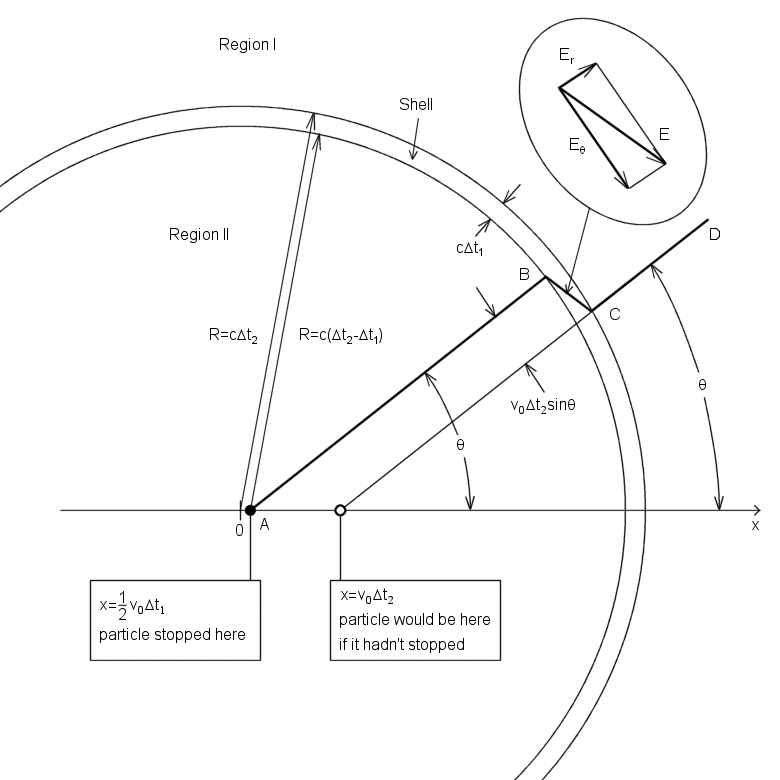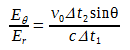# Radiation by an accelerated charge

Alternating magnetic field, electromagnetic radiation, electromagnetic induction

An electric field moves with the velocity that the particle had while emitting that field.Electric field of a charge that stops

Suppose a charge q has been moving at constant speed v0 in the x direction for a long time. Suddenly it stops after a short period of constant deceleration. Electric field of a charge travels radially outward and moves with the velocity that the charge had while emitting that field.

The velocity versus time graphThe electric field

Assuming that the v0 ≪ c, we can neglect the relativistic compression of the field lines. Time t=0 it was the moment when deceleration began, and position x=0 it was the position of the particle at that moment. The particle has been moved a little farther on before coming to a stop, Δx=1/2*v0Δt1. That distance is very small compared with the other distances in the picture.

We now examine the electric field at a time t=Δt2≫Δt1. The electric field reaches a distance R=cΔt2. Thus, the field at a distance greater than R=cΔt2, in region I must be a field of a charge which has been moving and is still moving at the constant speed v0. That field appears to come from the point x=v0Δt2 on the x axis. That is where the particle would be now if it hadn't stopped. On the other hand, the field at a distance less than c(Δt2-Δt1), in region II must be a field of a charge at rest close to the position x=0 (exactly at x=1/2*v0Δt1).What must the field be like in the transition region, the spherical shell of thickness cΔt1 between region I and region II? A field line segment AB lies on a cone around the x axis which includes a certain amount of flux from the charge q. Due to the Gauss' law, if CD makes the same angle θ with the axis, the cone on which it lies includes that same amount of flux. (Because the v0 ≪ c, we can neglect the relativistic compression of the field lines.) That's why AB and CD must be parts of the same field line, connected by a segment BC. The line segment BC shows us the direction of the filed E within the shell. This field E within the shell has both a radial component Er and a transverse component Eθ. From the geometry of the figure their ratio is easily found.(1)

Due to the Gauss' law, Er must have the same value within the shell thickness that it does in region II near B. That's why Er=q/4πε0R2=q/4πε0c2Δt22, and substituting this in Eq. 1 we obtain(2)
And v0/Δt1 = a, the magnitude of the (negative) acceleration, and cΔt2= R, so our result can be written(3)
A remarkable fact is here revealed: Eθ is proportional to 1/R, not to 1/R2! As time goes on and R increases, the transverse field Eθ will eventually become very much stronger than Er. Accompanying this transverse (that is, perpendicular to R) electric field will be a magnetic field of equal strength perpendicular to both R and E. This is a general property of an electromagnetic wave.

We now calculate the energy stored in the transverse electric field above, in the whole spherical shell. The energy density is(4)
The volume of the shell is 4πR2cΔt1, and the average value of sin2θ over a sphere is 2/3. The total energy of the transverse electric field is consequently(5)
To this we must add an equal amount for the energy stored in the transverse magnetic field:
Total energy in transverse electromagnetic field is(6)
The radius R has canceled out. This amount of energy simply travels outward, undiminished, with speed c from the site of the deceleration. Since Δt1 is the duration of the deceleration, and is also the duration of the electromagnetic pulse a distant observer measures, we can say that the power radiated during the acceleration process was(7)
As it is the square of the instantaneous acceleration that appears in Eq. 7, it doesn't matter whether a is positive or negative. Of course it ought not to, for stopping in one inertial frame could be starting in another. Speaking of different frames, Prad itself turns out to be Lorentz-invariant, which is sometimes very handy. That is because Prad is energy/time, and energy transforms like time, each being the fourth component of a four-vector. We have here a more general result than we might have expected. Equation 7 correctly gives the instantaneous rate of radiation of energy by a charged particle moving with variable acceleration-for instance, a particle vibrating in simple harmonic motion. It applies to a wide variety of radiating systems from radio antennas to atoms and nuclei.

radiation, accelerated, charge, electromagnetic, induction, wave, waves, physics, science,

Radiating Charge simulation - PhET INTERACTIVE SIMULATIONS

Purcell appendix B in the CGS system